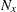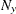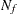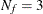# The PLS Procedure

### OUTPUT Statement

`OUTPUT` OUT=SAS-data-set keyword=names <…keyword=names> ;

You use the OUTPUT statement to specify a data set to receive quantities that can be computed for every input observation, such as extracted factors and predicted values. The following keywords are available:

PREDICTED

predicted values for responses

YRESIDUAL

residuals for responses

XRESIDUAL

residuals for predictors

XSCORE

extracted factors (X-scores, latent vectors, latent variables, T)

YSCORE

extracted responses (Y-scores, U)

STDY

standardized (centered and scaled) responses

STDX

standardized (centered and scaled) predictors

H

approximate leverage

PRESS

approximate predicted residuals

TSQUARE

scaled sum of squares of score values

STDXSSE

sum of squares of residuals for standardized predictors

STDYSSE

sum of squares of residuals for standardized responses

Suppose that there arepredictors andresponses and that the model hasselected factors.

• The keywords XRESIDUAL and STDX define an output variable for each predictor, sonames are required after each one.

• The keywords PREDICTED, YRESIDUAL, STDY, and PRESS define an output variable for each response, sonames are required after each of these keywords.

• The keywords XSCORE and YSCORE specify an output variable for each selected model factor. For these keywords, you provide only one base name, and the variables corresponding to each successive factor are named by appending the factor number to the base name. For example, if, then a specification of XSCORE=T would produce the variables T1, T2, and T3.

• Finally, the keywords H, TSQUARE, STDXSSE, and STDYSSE each specify a single output variable, so only one name is required after each of these keywords.